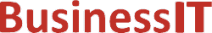O nás     Inzerce     KontaktSpolehlivé informace o IT již od roku 2011[Linux manuál]

# y0, y1, yn: Besselovy funkce druhého druhu

Originální popis anglicky: y0, y1, yn - Bessel functions of the second kind

Návod, kniha: POSIX Programmer's Manual

## STRUČNĚ

#include <math.h>

double y0(double x);

double y1(double x);

double yn(int n, double x);

## POPIS / INSTRUKCE

The y0(), y1(), and yn() functions shall compute Bessel functions of x of the second kind of orders 0, 1, and n, respectively.
An application wishing to check for error situations should set errno to zero and call feclearexcept(FE_ALL_EXCEPT) before calling these functions. On return, if errno is non-zero or fetestexcept(FE_INVALID | FE_DIVBYZERO | FE_OVERFLOW | FE_UNDERFLOW) is non-zero, an error has occurred.

## NÁVRATOVÁ HODNOTA

Upon successful completion, these functions shall return the relevant Bessel value of x of the second kind.
If x is NaN, NaN shall be returned.
If the x argument to these functions is negative, -HUGE_VAL or NaN shall be returned, and a domain error may occur.
If x is 0.0, -HUGE_VAL shall be returned and a range error may occur.
If the correct result would cause underflow, 0.0 shall be returned and a range error may occur.
If the correct result would cause overflow, -HUGE_VAL or 0.0 shall be returned and a range error may occur.

## CHYBY / ERRORY

These functions may fail if:
Domain Error
The value of x is negative.
If the integer expression (math_errhandling & MATH_ERRNO) is non-zero, then errno shall be set to [EDOM]. If the integer expression (math_errhandling & MATH_ERREXCEPT) is non-zero, then the invalid floating-point exception shall be raised.
Range Error
The value of x is 0.0, or the correct result would cause overflow.
If the integer expression (math_errhandling & MATH_ERRNO) is non-zero, then errno shall be set to [ERANGE]. If the integer expression (math_errhandling & MATH_ERREXCEPT) is non-zero, then the overflow floating-point exception shall be raised.
Range Error
The value of x is too large in magnitude, or the correct result would cause underflow.
If the integer expression (math_errhandling & MATH_ERRNO) is non-zero, then errno shall be set to [ERANGE]. If the integer expression (math_errhandling & MATH_ERREXCEPT) is non-zero, then the underflow floating-point exception shall be raised.

The following sections are informative.

None.

## APPLICATION USAGE

On error, the expressions (math_errhandling & MATH_ERRNO) and (math_errhandling & MATH_ERREXCEPT) are independent of each other, but at least one of them must be non-zero.

None.

None.

## SOUVISEJÍCÍ

feclearexcept() , fetestexcept() , isnan() , j0() , the Base Definitions volume of IEEE Std 1003.1-2001, Section 4.18, Treatment of Error Conditions for Mathematical Functions, <math.h> Portions of this text are reprinted and reproduced in electronic form from IEEE Std 1003.1, 2003 Edition, Standard for Information Technology -- Portable Operating System Interface (POSIX), The Open Group Base Specifications Issue 6, Copyright (C) 2001-2003 by the Institute of Electrical and Electronics Engineers, Inc and The Open Group. In the event of any discrepancy between this version and the original IEEE and The Open Group Standard, the original IEEE and The Open Group Standard is the referee document. The original Standard can be obtained online at http://www.opengroup.org/unix/online.html .
 2003 IEEE/The Open Group
©2011-2020 BusinessIT.cz, ISSN 1805-0522 | Názvy použité v textech mohou být ochrannými známkami příslušných vlastníků.
Provozovatel: Bispiral, s.r.o., kontakt: BusinessIT(at)Bispiral.com | Inzerce: Best Online Media, s.r.o., zuzana@online-media.cz
O vydavateli | Pravidla webu BusinessIT.cz a ochrana soukromí | pg(8688)